# The 6 Trig Ratios

For any right triangle, there are six trig ratios: Sine (sin), cosine (cos), tangent (tan), cosecant (csc), secant (sec), and cotangent (cot).

Here are the formulas for these six trig ratios: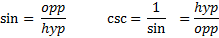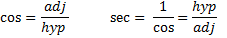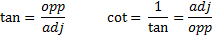Given a triangle, you should be able to identify all 6 ratios for all the angles (except the right angle).

Let's start by finding all 6 ratios for angle A.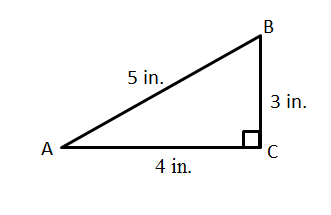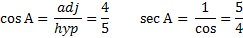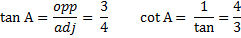Notice that the csc, sec, and cot can be found simply by flipping the ratio they are associated with. Or,
you can use the formulas.

To find the 6 ratios for angle B, just start over again and rethink them looking at angle B instead of angle A. This means that the opposite and the adjacent sides switch while the hypotenuse stays the same. Here are the 6 ratios for angle B: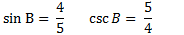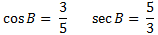Go back and compare the ratios from angle A with the ratios from angle B. You will notice quite a few relationships.

Practice: Find the following:1. Sin A
2. Cos A
3. Tan A
4. Csc A
5. Sec A
6. Cot A
7. Sin B
8. Cos B
9. Tan B
10. Csc B
11. Sec B
12. Cot B

Answers:Related Links: Math Fractions Factors